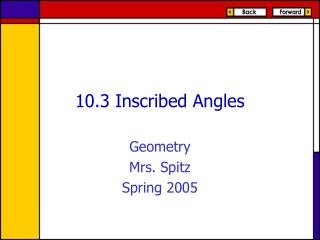DownloadDownload Presentation10.3 Inscribed Angles

# 10.3 Inscribed Angles

Télécharger la présentation## 10.3 Inscribed Angles

- - - - - - - - - - - - - - - - - - - - - - - - - - - E N D - - - - - - - - - - - - - - - - - - - - - - - - - - -
##### Presentation Transcript

1. 10.3 Inscribed Angles Geometry Mrs. Spitz Spring 2005

2. Objectives/Assignment • Reminder Quiz after this section. • Use inscribed angles to solve problems. • Use properties of inscribed polygons. • Assignment: pp. 616-617 #2-29 all

3. An inscribed angle is an angle whose vertex is on a circle and whose sides contain chords of the circle. The arc that lies in the interior of an inscribed angle and has endpoints on the angle is called the intercepted arc of the angle. Using Inscribed Angles

4. Theorem 10.8: Measure of an Inscribed Angle • If an angle is inscribed in a circle, then its measure is one half the measure of its intercepted arc. mADB = ½m

5. m = 2mQRS = 2(90°) = 180° Ex. 1: Finding Measures of Arcs and Inscribed Angles • Find the measure of the blue arc or angle.

6. Ex. 1: Finding Measures of Arcs and Inscribed Angles • Find the measure of the blue arc or angle. m = 2mZYX = 2(115°) = 230°

7. Ex. 1: Finding Measures of Arcs and Inscribed Angles • Find the measure of the blue arc or angle. 100° m = ½ m ½ (100°) = 50°

8. Ex. 2: Comparing Measures of Inscribed Angles • Find mACB, mADB, and mAEB. The measure of each angle is half the measure of m = 60°, so the measure of each angle is 30°

9. If two inscribed angles of a circle intercept the same arc, then the angles are congruent. C  D Theorem 10.9

10. Ex. 3: Finding the Measure of an Angle • It is given that mE = 75°. What is mF? • E and F both intercept , so E  F. So, mF = mE = 75° 75°

11. Theater Design. When you go to the movies, you want to be close to the movie screen, but you don’t want to have to move your eyes too much to see the edges of the picture. Ex. 4: Using the Measure of an Inscribed Angle

12. If E and G are the ends of the screen and you are at F, mEFG is called your viewing angle. Ex. 4: Using the Measure of an Inscribed Angle

13. You decide that the middle of the sixth row has the best viewing angle. If someone else is sitting there, where else can you sit to have the same viewing angle? Ex. 4: Using the Measure of an Inscribed Angle

14. Solution: Draw the circle that is determined by the endpoints of the screen and the sixth row center seat. Any other location on the circle will have the same viewing angle. Ex. 4: Using the Measure of an Inscribed Angle

15. If all of the vertices of a polygon lie on a circle, the polygon is inscribed in the circle and the circle is circumscribed about the polygon. The polygon is an inscribed polygon and the circle is a circumscribed circle. Using Properties of Inscribed Polygons

16. If a right triangle is inscribed in a circle, then the hypotenuse is a diameter of the circle. Conversely, if one side of an inscribed triangle is a diameter of the circle, then the triangle is a right triangle and the angle opposite the diameter is the right angle. B is a right angle if and only if AC is a diameter of the circle. Theorem 10.10

17. A quadrilateral can be inscribed in a circle if and only if its opposite angles are supplementary. D, E, F, and G lie on some circle, C, if and only if mD + mF = 180° and mE + mG = 180° Theorem 10.11

18. Find the value of each variable. AB is a diameter. So, C is a right angle and mC = 90° 2x° = 90° x = 45 Ex. 5: Using Theorems 10.10 and 10.11 2x°

19. Find the value of each variable. DEFG is inscribed in a circle, so opposite angles are supplementary. mD + mF = 180° z + 80 = 180 z = 100 Ex. 5: Using Theorems 10.10 and 10.11 z° 120° 80° y°

20. Find the value of each variable. DEFG is inscribed in a circle, so opposite angles are supplementary. mE + mG = 180° y + 120 = 180 y = 60 Ex. 5: Using Theorems 10.10 and 10.11 z° 120° 80° y°

21. In the diagram, ABCD is inscribed in circle P. Find the measure of each angle. ABCD is inscribed in a circle, so opposite angles are supplementary. 3x + 3y = 180 5x + 2y = 180 Ex. 6: Using an Inscribed Quadrilateral 2y° 3y° 3x° 2x° To solve this system of linear equations, you can solve the first equation for y to get y = 60 – x. Substitute this expression into the second equation.

22. 5x + 2y = 180. 5x + 2 (60 – x) = 180 5x + 120 – 2x = 180 3x = 60 x = 20 y = 60 – 20 = 40 Ex. 6: Using an Inscribed Quadrilateral Write the second equation. Substitute 60 – x for y. Distributive Property. Subtract 120 from both sides. Divide each side by 3. Substitute and solve for y. x = 20 and y = 40, so mA = 80°, mB = 60°, mC = 100°, and mD = 120°

23. Reminder: • Quiz after this section. • Quiz after 10.5